×#### Thank you for registering.

One of our academic counsellors will contact you within 1 working day.

Click to Chat

1800-1023-196

+91-120-4616500

CART 0

• 0

MY CART (5)

Use Coupon: CART20 and get 20% off on all online Study Material

ITEM
DETAILS
MRP
DISCOUNT
FINAL PRICE
Total Price: Rs.

There are no items in this cart.
Continue Shopping• Complete JEE Main/Advanced Course and Test Series
• OFFERED PRICE: Rs. 15,900
• View Details

```Revision Notes on Polynomials

Polynomial

Polynomial is an algebraic expression which includes constants, variables and exponents. It is the expression in which the variables have only positive integral powers.

Example

1. 4x3 + 3x2 + x +3 is a polynomial in variable x.

2. 4x2 + 3x-1 - 4 is not a polynomial as it has negative power.

3. 3x3/2 + 2x – 3 is not a polynomial.Polynomials are denoted by p(x), q(x) etc.

In the above polynomial 2x2, 3y and 2 are the terms of the polynomial.

2 and 3 are the coefficient of the x2 and y respectively.

x and y are the variables.

2 is the constant term which has no variable.

Polynomials in One Variable

If there is only one variable in the expression then this is called the polynomial in one variable.

Example

x3 + x – 4 is polynomial in variable x and is denoted by p(x).

r2 + 2 is polynomial in variable r and is denoted by p(r).

Types of polynomials on the basis of the number of terms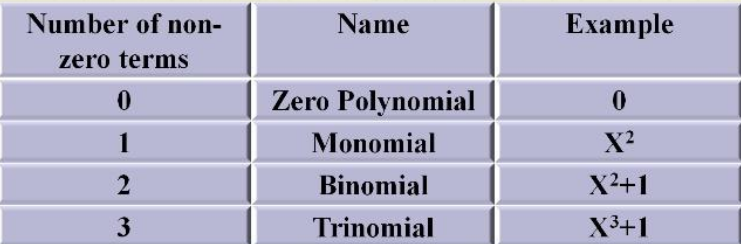Types of polynomials on the basis of the number of degrees

The highest value of the power of the variable in the polynomial is the degree of the polynomial.Zeroes of a Polynomial

If p(x) is a polynomial then the number ‘a’ will be the zero of the polynomial with p(a) = 0. We can find the zero of the polynomial by equating it to zero.

Example: 1

Given polynomial is p(x) = x - 4

To find the zero of the polynomial we will equate it to zero.

x - 4 = 0

x = 4

p(4) = x – 4 = 4 – 4 = 0

This shows that if we place 4 in place of x, we got the value of the polynomial as zero. So 4 is the zero of this polynomial. And also we are getting the value 4 by equating the polynomial by 0.

So 4 is the zero of the polynomial or root of the polynomial.

The root of the polynomial is basically the x-intercept of the polynomial.If the polynomial has one root, it will intersect the x-axis at one point only and if it has two roots then it will intersect at two points and so on.

Example: 2

Find p (1) for the polynomial p (t) = t2 – t + 1

p (1) = (1)2 – 1 + 1

= 1 – 1 + 1

= 1

Remainder Theorem

We know the property of division which follows in the basic division, i.e.

Dividend = (Divisor × Quotient) + Remainder

This same follows the division of polynomial.

If p(x) and g(x) are two polynomials in which the degree of p(x) ≥ degree of g(x) and g(x) ≠ 0 are given then we can get the q(x) and r(x) so that:

P(x) = g(x) q(x) + r(x),

where r(x) = 0 or degree of r(x) < degree of g(x).

It says that p(x) divided by g(x), gives q(x) as quotient and r(x) as remainder.

Let’s understand it with an example

Division of a Polynomial with a MonomialWe can see that ‘x’ is common in the above polynomial, so we can write it as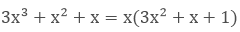Hence 3x2 + x + 1 and x the factors of 3x3 + x2 + x.

Steps of the Division of a Polynomial with a Non –Zero Polynomial

Divide x2 - 3x -10 by 2 + x

Step 1:  Write the dividend and divisor in the descending order i.e. in the standard form. x2 - 3x -10 and x + 2

Divide the first term of the dividend with the first term of the divisor.

x2/x = x this will be the first term of the quotient.

Step 2: Now multiply the divisor with this term of the quotient and subtract it from the dividend.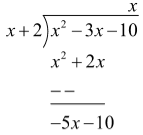Step 3: Now the remainder is our new dividend so we will repeat the process again by dividing the dividend with the divisor.

Step 4: – (5x/x) = – 5

Step 5: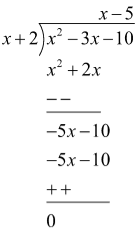The remainder is zero.

Hence x2 - 3x – 10 = (x + 2)(x - 5) + 0

Dividend = (Divisor × Quotient) + Remainder

Remainder Theorem says that if p(x) is any polynomial of degree greater than or equal to one and let ‘t’ be any real number and p (x) is divided by the linear polynomial x – t, then the remainder is p(t).

As we know that

P(x) = g(x) q(x) + r(x)

If p(x) is divided by (x-t) then

If x = t

P (t) = (t - t).q (t) + r = 0

To find the remainder or to check the multiple of the polynomial we can use the remainder theorem.

Example:

What is the remainder if a4 + a3 – 2a2 + a + 1 is divided by a – 1.

Solution:

P(x) = a4 + a3 – 2a2 + a + 1

To find the zero of the (a – 1) we need to equate it to zero.

a -1 = 0

a = 1

p (1) = (1)4 + (1)3 – 2(1)2 + (1) + 1

= 1 + 1 – 2 + 1 + 1

= 2

So by using the remainder theorem, we can easily find the remainder after the division of polynomial.

Factor Theorem

Factor theorem says that if p(y) is a polynomial with degree n≥1 and t is a real number, then

(y - t) is a factor of p(y), if p(t) = 0, and

P (t) = 0 if (y – t) is a factor of p(y).

Example: 1

Check whether g(x) = x – 3 is the factor of p(x) = x3 - 4x2 + x + 6 using factor theorem.

Solution:

According to the factor theorem if x - 3 is the factor of p(x) then p(3) = 0, as the root of x – 3 is 3.

P (3) = (3)3 - 4(3)2 + (3) + 6

= 27 – 36 + 3 + 6 = 0

Hence, g(x) is the factor of p(x).

Example: 2

Find the value of k, if x – 1 is a factor of p(x) = kx2 – √2x + 1

Solution:

As x -1 is the factor so p(1) = 0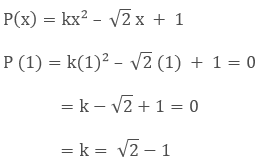Factorization of Polynomials

Factorization can be done by three methods

1. By taking out the common factor

If we have to factorize x2 –x then we can do it by taking x common.

x(x – 1) so that x and x-1 are the factors of x2 – x.

2. By grouping

ab + bc + ax + cx = (ab + bc) + (ax + cx)

= b(a + c) + x(a + c)

= (a + c)(b + x)

3. By splitting the middle term

x2 + bx + c = x2 + (p + q) + pq

= (x + p)(x + q)

This shows that we have to split the middle term in such a way that the sum of the two terms is equal to ‘b’ and the product is equal to ‘c’.

Example: 1

Factorize 6x2 + 17x + 5 by splitting the middle term.

Solution:

If we can find two numbers p and q such that p + q = 17 and pq = 6 × 5 = 30, then we can get the factors.

Some of the factors of 30 are 1 and 30, 2 and 15, 3 and 10, 5 and 6, out of which 2 and 15 is the pair which gives p + q = 17.

6x2 + 17x + 5 =6 x2 + (2 + 15) x + 5

= 6 x2 + 2x + 15x + 5

= 2 x (3x + 1) + 5(3x + 1)

= (3x + 1) (2x + 5)

Algebraic Identities

1. (x + y)2 = x2 + 2xy + y2

2. (x - y)2 = x2 - 2xy + y2

3. (x + y) (x - y) = x2 - y2

4. (x + a) (x + b) = x2 + (a + b)x + ab

5. (x + y + z)2 = x2 + y2 + z2 + 2xy + 2yz + 2zx

6. (x + y)3 = x3 + y3 + 3xy(x + y) = x3+ y3 + 3x2y + 3xy2

7. (x - y)3 = x3- y3 - 3xy(x - y) = x3 - y3 - 3x2y + 3xy2

8. x3 + y3 = (x + y)(x2 – xy + y2)

9. x3 - y3 = (x - y)(x2 + xy + y2)

10. x3 + y3 + z3 - 3xyz = (x + y + z)(x2 + y2 + z2 – xy – yz - zx)
x3 + y3 + z3 = 3xyz if x + y + z = 0

Example: 2

Factorize 8x3 + 27y3 + 36x2y + 54xy2

Solution:

The given expression can be written as

= (2x)3 + (3y)3 + 3(4x2) (3y) + 3(2x) (9y2)

= (2x)3 + (3y)3 + 3(2x)2(3y) + 3(2x)(3y)2

= (2x + 3y)3 (Using Identity VI)

= (2x + 3y) (2x + 3y) (2x + 3y) are the factors.

Example: 3

Factorize 4x2 + y2 + z2 – 4xy – 2yz + 4xz.

Solution:

4x2 + y2 + z2 – 4xy – 2yz + 4xz = (2x)2 + (–y)2 + (z)2 + 2(2x) (-y)+ 2(–y)(z) + 2(2x)(z)

= [2x + (- y) + z]2 (Using Identity V)

= (2x – y + z)2 = (2x – y + z) (2x – y + z)
```### Course Features

• 728 Video Lectures
• Revision Notes
• Previous Year Papers
• Mind Map
• Study Planner
• NCERT Solutions
• Discussion Forum
• Test paper with Video Solution